# Complement

In previous lessons, we learned that a set is a group of objects, and that Venn diagrams can be used to illustrate both set relationships and logical relationships.

Example 1: Given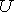= {students who attend The Kewl School} and  A = {students in Mrs. Glosser's class}. What is the set of all students who attend The Kewl School that are not in Mrs. Glosser's class?

Analysis: The relationship between these sets is illustrated in the Venn diagram below.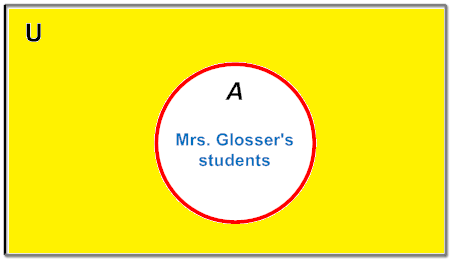Answer: The shaded area outside A represents all students who attend The Kewl School that are not in Mrs. Glosser's class.

In example 1, the shaded area represents the complement of Set A. The complement of A, denoted by A', consists of all students in The Kewl school that are not in Mrs. Glosser's class. Recall that a Universal Set is the set of all elements under consideration, denoted by capital, and that all other sets are subsets of the Universal Set. Now we can define the complement of a set.

Definition: The complement of a set A, denoted by A', is the set of elements which belong tobut which do not belong to A.

The complement of set A is denoted by A', You can also say "complement of A in", or "A-prime". We can now label the sets in example 1 using this notation.

Example 1: Given= {students who attend The Kewl School} and  A = {students in Mrs. Glosser's class}. What is the set of all students who attend The Kewl School that are not in Mrs. Glosser's class?

Analysis: The relationship between these sets is illustrated in the Venn diagram below.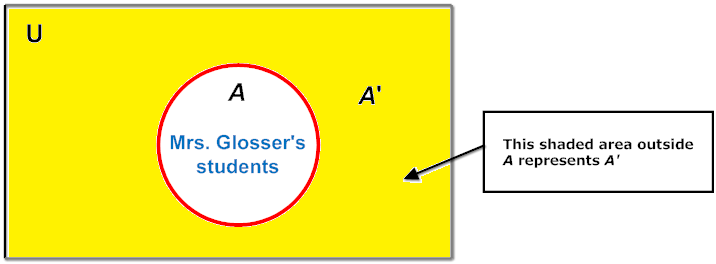Answer: The shaded area outside A represents A', which is all students who attend The Kewl School that are not in Mrs. Glosser's class.

Another way to think of the complement of a set is as follow: Given set A, the complement of A is the set of all elements in the universal set, that are not in A. Using set-builder notation, we can write:

A= { x | x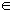and xA }

Let's find the complement of a set of numbers.

Example 2: Given= {single digits} and  B = {0, 1, 4, 5, 6, 7, 8}, find the complement of B.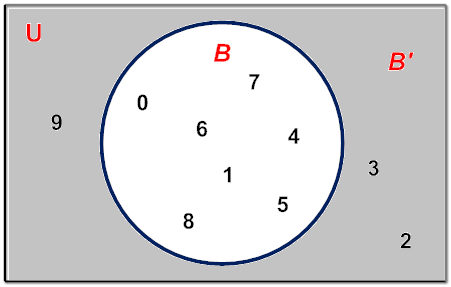Thus B is the set of all numbers inthat are not in B. Using set-builder notation, we can write: B= { x | xand xB }

In examples 3 through 5, the universal set is the English alphabet.

Example 3: Given= {a, b, c, ..., x, y, z} and  X = {a, b, c, d, e}, find X'.

Analysis: X' would consist of all letters in the English alphabet that are not in X. This is shown in the Venn Diagram below.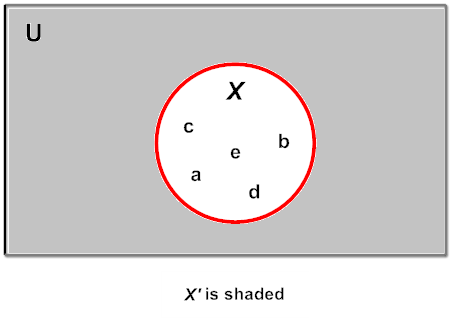Answer: X= {f, g, h, ..., x, y, z}

Example 4: Given= {a, b, c, ..., x, y, z}, X = {a, b, c, d, e} and  Y = {e, f, g}, find Y'.

Analysis: Y' would consist of all letters in the English alphabet that are not in Y. This is shown in the Venn Diagram below..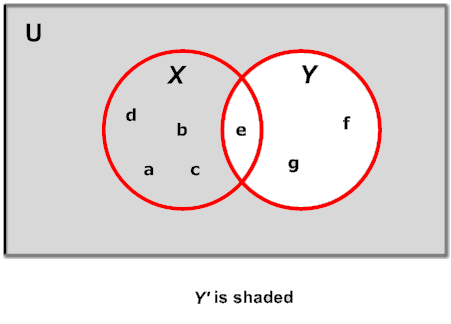Answer: Y= {a, b, c, d, h, i, j, ..., x, y, z}

Example 5: Given= {a, b, c, ..., x, y, z}, P = {a, b, c, d, e} and  Q = {x, y, z}, find Q'.

Analysis: Q' consists of all the letters in the alphabet that are not in QThis is shown in the Venn Diagram below..Answer: Q= {a, b, c, d, e, f, g, h ..., u, v, w}

Looking at the examples above, a set and its complement have no elements in common. The union of a set and its complement is the Universal Set. The intersection of a set and its complement is the null set. These statements are summarized below:

## Tips:There are several different ways to represent the complement of a set, as shown below.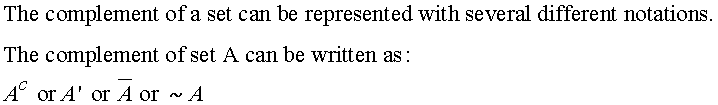All of these notations have the same meaning. However, for the purpose of this instructional unit, we have chosen to use A', read as A-prime.

Let's look at some examples of complement that involve set-builder notation and infinite sets.

Example 6: If= { n | n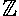and -6 < n < 7 } and  B = { y | y even number; -5 < y < 6 }, then what is the complement of B?Answer: B= {-5, -3, -1, 1, 3, 5, 6}

Example 7: Given= {counting numbers > 1} and  C = {prime numbers}, find C'.

Analysis: C' would consist of all counting numbers greater than 1 that are not primeThis is shown in the Venn Diagram below.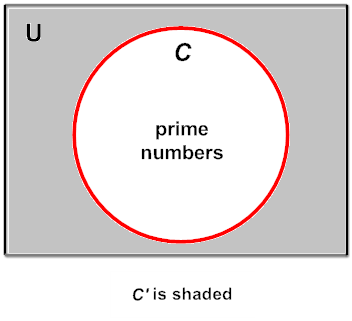Summary: Given set A, the complement of A is the set of all element in the universal set, that are not in A. The complement of set A is denoted as A' and is read as A-prime. The formal definition of complement is shown below.

A= { x | xand xA }

### Exercises

 1. If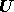= {students who attend a school} and X' = {students who do not ride the bus}, then which of the following is setX? X = {students who do not ride their bikes} X = {students who do not walk} X = {students who ride the bus} None of the above. RESULTS BOX:
 2. If= { k | k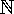and 1 ≤ K ≤ 10 } and P = {1, 3, 5, 7, 9}, then which of the following is the complement of set P? P' = {2, 4, 6, 8, 10} P' = {0, 2, 4, 6, 8} P' = {0, 2, 4, 6, 8, 10} All of the above. RESULTS BOX:
 3. Given= {a, b, c, ..., x, y, z}, M = {a, b, c} and N = {c, d, e}, find N'. N'  = {a, b, c, d, e} N' = {a, b, f, g, h, i, j, ..., x, y, z} N'  = {a, b, c, ..., x, y, z} None of the above. RESULTS BOX:
 4. If= { n | -7 < n <  8 } and P = {-6, -4, -2, 4, 6}, then which of the following is the complement of set P? P' = {-5, -3, -1, 1, 3, 4, 5, 6, 7} P' = {-5, -3, -1, 0, 1, 2, 3, 4, 5, 7} P' = {-5, -3, -1, 0, 1, 2, 3, 5, 7} All of the above RESULTS BOX:
 5. If= {whole numbers} and A' = {odd whole numbers}, then which of the following is set A? A = Ø A = {composite numbers} A = {prime numbers} A = {even whole numbers} RESULTS BOX: